# Find disjoint sets in a graph using disjoint set ADT operations FIND, UNION

In this article, we are going to see usage of disjoint set ADT operation to find disjoint sets in a graph.
Submitted by Radib Kar, on September 11, 2020

Let us first discuss what disjoint sets in a graph is? Disjoint sets in a graph mean components of a graph. Each connected component is treated as a disjoint set since it has no relation with the other components. Each connection (edge) is said to be the relation between two nodes. Two nodes having a relation falls in the same set.

In the below input graph,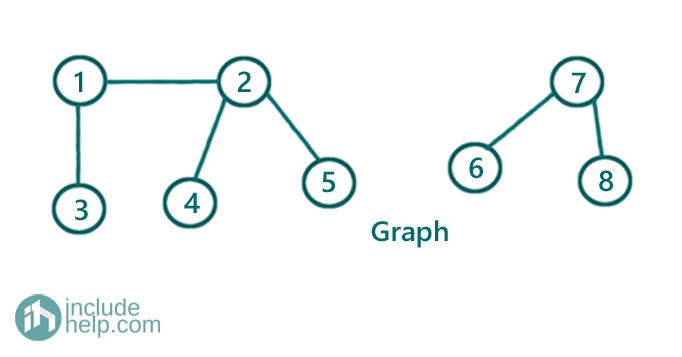We have two components and thus two disjoint sets. Those are,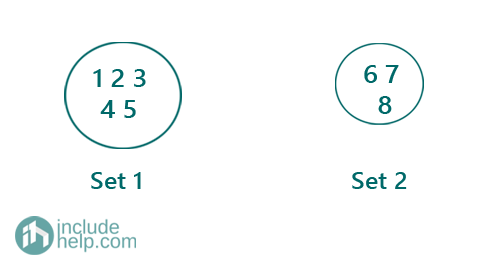Now, how we can find the disjoint sets. Here comes the find(X) & UNION (X,Y) ADT operation.

The operation finds the set name and here we use array for that. We use a parent array to store all set names initially. Initially all are themselves (i for node i).

We call it a parent array to store the set names: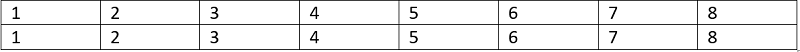The algorithm is:

For each edge in the edge list:

If the nodes on the edge are in same set, do nothing, otherwise, we will UNION them as they have relations between them and should come under same set.

To check whether the two nodes are in same set we use FIND

Implementation of FIND & UNION

1) FIND(X)

We use a recursive function find (X, parent []) to find the set name of element X.

The implementation is like below:

```FUNCTION find(int X, int parent[]):
If(parent[x]==X)
return X;
return find(parent[x], parent)
End FUNCTION
```

2) UNION (X, Y)

Union means we bring both elements under same set which is as simple assigning X as parent of Y

parent[Y]=X

C++ Implementation:

```#include <bits/stdc++.h>
using namespace std;

//FIND(X)
int find(int X, vector<int>& parent)
{
if (parent[X] == X)
return X;

return find(parent[X], parent);
}

//UNION(X,Y)
void UNION(int X, int Y, vector<int>& parent)
{
parent[Y] = X;
}

int main()
{
int n, m;

cout << "Enter number of edges\n";
cin >> n;
cout << "Enter number of nodes\n";
cin >> m;

//edges list
//each edge is a vector [u,v]
vector<vector<int> > edges(n);

for (int i = 0; i < n; i++) {
int u, v;
cout << "info for edge " << i + 1 << endl;
cout << "Enter source node u: ";
cin >> u;
cout << "Enter destination node v: ";
cin >> v;
edges[i] = vector<int>{ u, v };
}

vector<int> parent(m + 1);

for (int i = 1; i <= m; i++)
parent[i] = i;

for (auto edge : edges) {
//find set name for source node, u
int x = find(edge, parent);
//cout<<x<<endl;
//find set name for destination node, v
int y = find(edge, parent);
//cout<<y<<endl;
//if they are not in same set already, do their union
if (x != y) {
UNION(x, y, parent);
}
}

//number of disjoint set is number of -1 in the parent set
int count = 0;
for (int i = 1; i <= m; i++)
if (parent[i] == i)
count++;
cout << "Number of disjoint set is :" << count << endl;

cout << "printing the parent array now:\n";
for (int i = 1; i <= m; i++)
cout << parent[i] << " ";
cout << endl;

return 0;
}
```

Output:

```Enter number of edges
6
Enter number of nodes
8
info for edge 1
Enter source node u: 1
Enter destination node v: 2
info for edge 2
Enter source node u: 1
Enter destination node v: 3
info for edge 3
Enter source node u: 2
Enter destination node v: 4
info for edge 4
Enter source node u: 2
Enter destination node v: 5
info for edge 5
Enter source node u: 6
Enter destination node v: 7
info for edge 6
Enter source node u: 7
Enter destination node v: 8
Numebr of disjoint set is :2
printing the parent array now:
1 1 1 1 1 6 6 6
```

Dry Run for the example:

So the edge list is:

[ [1, 2],

[1, 3],

[2, 4],

[2, 5],

[6, 7],

[7, 8] ]

We will process each edge one by one and do necessary FIND & UNION

Initially parent[] is: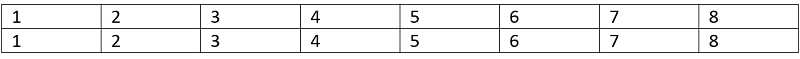Edge 1:

So source is 1 and destination is 2

We will find parent of both 1 & 2

Finding parent of 1

parent is 1 so it returns 1

Finding parent of 2

parent is 2 so it returns 2

Since both their set names( parent) are different we do a union

Thus parent=1 now, so after processing edge 1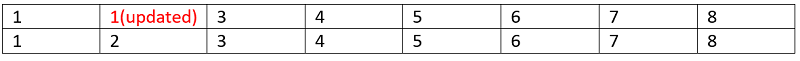Edge 2:

So source is 1 and destination is 3

We will find parent of both 1 & 3

Finding parent of 1

parent is 1 so it returns 1

Finding parent of 3

parent is 3 so it returns 3

Since both their set names( parent) are different we do a union

Thus parent=1 now, so after processing edge 2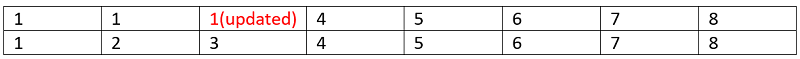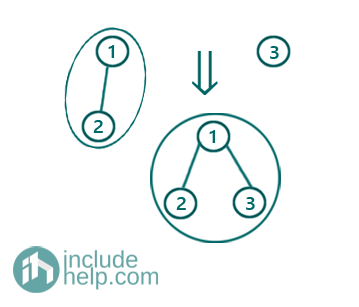Edge 3:

So source is 2 and destination is 4

We will find parent of both 2 & 4

Finding parent of 2

parent is 1 so it returns find(1, parent) and that returns 1 ultimately (parent== 1)

Finding parent of 4

parent is 4 so it returns 4

Since both their set names( parent) are different we do a union

Thus parent=1 now, so after processing edge 3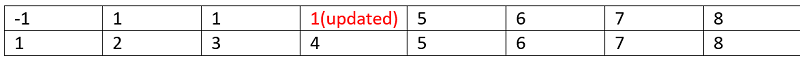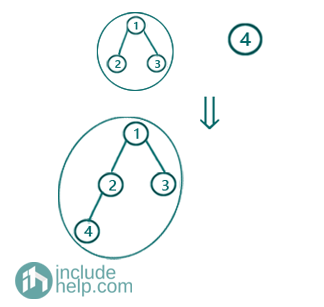Edge 4:

So source is 2 and destination is 5

We will find parent of both 2 & 5

Finding parent of 2

parent is 1 so it returns find(1, parent) and that returns 1 ultimately (parent== 1)

Finding parent of 5

parent is 5 so it returns 5

Since both their set names( parent) are different we do a union

Thus parent=1 now, so after processing edge 4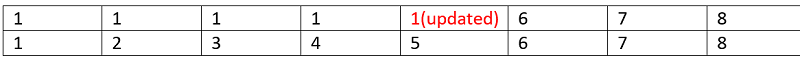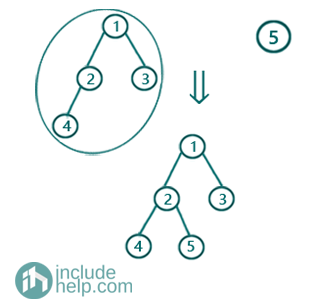Edge 5:

So source is 6 and destination is 7

We will find parent of both 6 & 7

Finding parent of 6

parent is 6 so it returns 6

Finding parent of 7

parent is 7 so it returns 7

Since both their set names( parent) are different we do a union

Thus parent=6 now, so after processing edge 5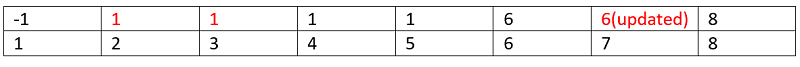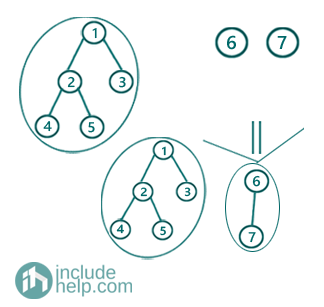Edge 6:

So source is 7 and destination is 8

We will find parent of both 7 & 8

Finding parent of 7

parent is 6 so it returns find(6, parents) which ultimately returns 6

Finding parent of 8

parent is 8 and thus it returns 8

Since both their set names( parent) are different we do a union

Thus parent=6 now, so after processing edge 6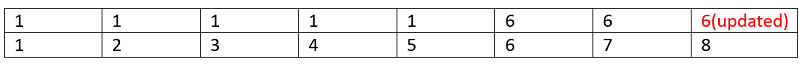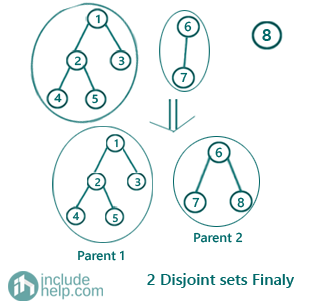So, the final parent array is: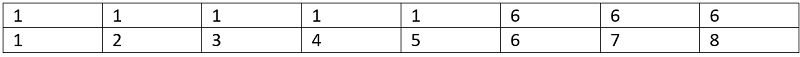Hence there are two disjoint sets with parents 1 & 6.

All nodes having same parent fall into same set. So the sets are: [1, 2, 3,4 5] & [6, 7, 8]

Languages: » C » C++ » C++ STL » Java » Data Structure » C#.Net » Android » Kotlin » SQL
Web Technologies: » PHP » Python » JavaScript » CSS » Ajax » Node.js » Web programming/HTML
Solved programs: » C » C++ » DS » Java » C#
Aptitude que. & ans.: » C » C++ » Java » DBMS
Interview que. & ans.: » C » Embedded C » Java » SEO » HR
CS Subjects: » CS Basics » O.S. » Networks » DBMS » Embedded Systems » Cloud Computing
» Machine learning » CS Organizations » Linux » DOS
More: » Articles » Puzzles » News/Updates